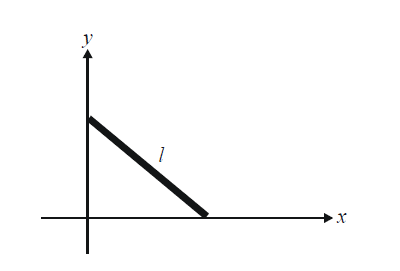# Basic Examples On Ellipses Set-6

Go back to  'Ellipse'

Example - 14

Consider a focal chord AB of the ellipse $$\frac{{{x^2}}}{{{a^2}}} + \frac{{{y^2}}}{{{b^2}}} = 1$$ where the eccentric angles of A and B are $${\theta _1}\;\text{and}\;{\theta _2}$$ respectively. If e is the eccentricity of the ellipse, prove that

$e = \frac{{\sin {\theta _1} + \sin {\theta _2}}}{{\sin ({\theta _1} + {\theta _2})}}$

Solution: The chord joining A and B has the equation

$\frac{x}{a}\cos \left( {\frac{{{\theta _1} + {\theta _2}}}{2}} \right) + \frac{y}{a}\sin \left( {\frac{{{\theta _1} + {\theta _2}}}{2}} \right) = \cos \left( {\frac{{{\theta _1} - {\theta _2}}}{2}} \right)\qquad\qquad...\left( 1 \right)$

Since AB is a focal chord of the ellipse (say, it passes through $${F_1}(ae,\,0)),$$the coordinates of the focus must satisfy (1) so that we have

$e\cos \left( {\frac{{{\theta _1} + {\theta _2}}}{2}} \right) = \cos \left( {\frac{{{\theta _1} - {\theta _2}}}{2}} \right)\\\qquad\qquad\qquad \qquad\qquad\quad e = \frac{{\cos \left( {\frac{{{\theta _1} - {\theta _2}}}{2}} \right)}}{{\cos \left( {\frac{{{\theta _1} + {\theta _2}}}{2}} \right)}}\quad\quad \quad...\left( 2 \right)$

Multiplying the numerator and denominator of the RHS of (2) by $$2\sin \left( {\frac{{{\theta _1} + {\theta _2}}}{2}} \right),$$ we have

\begin{align}&e = \frac{{2\cos \left( {\frac{{{\theta _1} - {\theta _2}}}{2}} \right)\sin \left( {\frac{{{\theta _1} + {\theta _2}}}{2}} \right)}}{{2\cos \left( {\frac{{{\theta _1} + {\theta _2}}}{2}} \right)\sin \left( {\frac{{{\theta _1} + {\theta _2}}}{2}} \right)}}\\&{\rm{ \;\;}} = \frac{{\sin {\theta _1} + \sin {\theta _2}}}{{\sin ({\theta _1} + {\theta _2})}}\end{align}

## INTERSECTION OF A LINE WITH AN ELLIPSE

Example – 15

Consider an ellipse $$\frac{{{x^2}}}{{{a^2}}} + \frac{{{y^2}}}{{{b^2}}} = 1$$ and a variable line $$y = mx + c.$$ What is the condition on m and c such that the line

(a) intersects the ellipse in two distinct points?

(b) touches the ellipse?

(c) does not intersect with the ellipse ?

Solution: As we’ve done in the case of circles and parabolas, to find the intersection (points) of the line and the ellipse, we must solve their equations simultaneously;

\begin{align}&\frac{{{x^2}}}{{{a^2}}} + \frac{{{y^2}}}{{{b^2}}} = 1 ;\,\,\,\,\,y = mx + c\\&\Rightarrow \quad\frac{{{x^2}}}{{{a^2}}} + \frac{{{{(mx + c)}^2}}}{{{b^2}}} = 1\\&\Rightarrow\quad ({a^2}{m^2} + {b^2}){x^2} + 2{a^2}mcx + {a^2}({c^2} - {b^2}) = 0\quad\qquad\qquad...\left( 1 \right)\end{align}

The line $$y = mx + c$$

(a) intersects the ellipse

(b) touches the ellipse

(c) does not touch / intersect the ellipse

accordingly, as the quadratic (1) has its discriminant greater than, equal to or less than 0.

The condition for tangency (D = 0) is of special intersect. Verify that it comes out to be

${c^2} = {a^2}{m^2} + {b^2}$

Thus, we can say that the line $$y = mx \pm \sqrt {{a^2}{m^2} + {b^2}}$$ will always be a tangent to the ellipse, whatever may be the value of m. We discuss tangents in more detail in the next section.

## TRY YOURSELF - I

Q. 1  Let P be a variable point on the ellipse $$\frac{{{x^2}}}{{{a^2}}} + \frac{{{y^2}}}{{{b^2}}} = 1$$ with foci S1 and S2. Find $$\max ({\rm{area}}(\Delta P{S_1}{S_2})).$$

Q. 2  Find the equation of the ellipse with foci at $$(0,\,\, \pm 4)$$ and eccentricity

Q. 3  Show that $${x^2} + 4{y^2} + 2x + 16y + 13 = 0$$ is the equation of an ellipse. Where are its foci?

Q. 4  Find the equation of the ellipse whose foci are $$( \pm 2,\,3)$$ and whose semi-minor axis is of length $$\sqrt 5 .$$

Q. 5  A straight rod of length $$l$$ slides between the x-axis and the y-axis, as shown. Show that the locus of its mid-point is an ellipse. What is its eccentricity?Q. 6  Show that the triangle with vertices (1, 2), (3, –1) and (–2, 1) lies completely inside the ellipse $${x^2} + 2{y^2} = 13.$$